Next: The torsion pendulum Up: Oscillatory motion Previous: Introduction

## Simple harmonic motion

Let us reexamine the problem of a mass on a spring (see Sect. 5.6). Consider a masswhich slides over a horizontal frictionless surface. Suppose that the mass is attached to a light horizontal spring whose other end is anchored to an immovable object. See Fig. 42. Letbe the extension of the spring: i.e., the difference between the spring's actual length and its unstretched length. Obviously,can also be used as a coordinate to determine the horizontal displacement of the mass.

The equilibrium state of the system corresponds to the situation where the mass is at rest, and the spring is unextended (i.e.,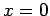). In this state, zero net force acts on the mass, so there is no reason for it to start to move. If the system is perturbed from this equilibrium state (i.e., if the mass is moved, so that the spring becomes extended) then the mass experiences a restoring force given by Hooke's law:(503)

Here,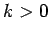is the force constant of the spring. The negative sign indicates thatis indeed a restoring force. Note that the magnitude of the restoring force is directly proportional to the displacement of the system from equilibrium (i.e.,). Of course, Hooke's law only holds for small spring extensions. Hence, the displacement from equilibrium cannot be made too large. The motion of this system is representative of the motion of a wide range of systems when they are slightly disturbed from a stable equilibrium state.

Newton's second law gives following equation of motion for the system: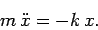(504)

This differential equation is known as the simple harmonic equation, and its solution has been known for centuries. In fact, the solution is(505)

where,, and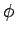are constants. We can demonstrate that Eq. (505) is indeed a solution of Eq. (504) by direct substitution. Substituting Eq. (505) into Eq. (504), and recalling from calculus thatand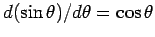, we obtain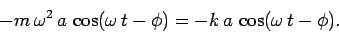(506)

It follows that Eq. (505) is the correct solution provided(507)

Figure 95 shows a graph ofversusobtained from Eq. (505). The type of motion shown here is called simple harmonic motion. It can be seen that the displacementoscillates between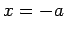and. Here,is termed the amplitude of the oscillation. Moreover, the motion is periodic in time (i.e., it repeats exactly after a certain time period has elapsed). In fact, the period is(508)

This result is easily obtained from Eq. (505) by noting that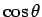is a periodic function ofwith period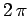. The frequency of the motion (i.e., the number of oscillations completed per second) is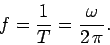(509)

It can be seen thatis the motion's angular frequency (i.e., the frequencyconverted into radians per second). Finally, the phase angledetermines the times at which the oscillation attains its maximum amplitude,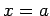: in fact,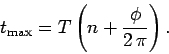(510)

Here,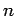is an arbitrary integer.

Table 4: Simple harmonic motion.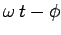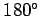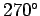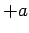00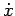0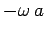0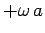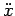00

Table 4 lists the displacement, velocity, and acceleration of the mass at various phases of the simple harmonic cycle. The information contained in this table can easily be derived from the simple harmonic equation, Eq. (505). Note that all of the non-zero values shown in this table represent either the maximum or the minimum value taken by the quantity in question during the oscillation cycle.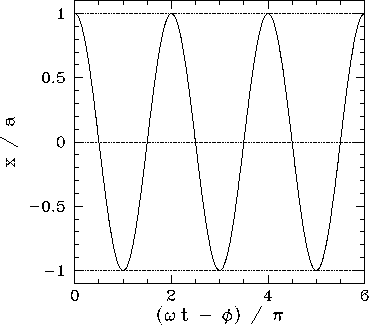We have seen that when a mass on a spring is disturbed from equilibrium it executes simple harmonic motion about its equilibrium state. In physical terms, if the initial displacement is positive () then the restoring force overcompensates, and sends the system past the equilibrium state () to negative displacement states (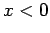). The restoring force again overcompensates, and sends the system back throughto positive displacement states. The motion then repeats itself ad infinitum. The frequency of the oscillation is determined by the spring stiffness,, and the system inertia,, via Eq. (507). In contrast, the amplitude and phase angle of the oscillation are determined by the initial conditions. Suppose that the instantaneous displacement and velocity of the mass at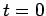areand, respectively. It follows from Eq. (505) that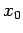(511)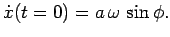(512)

Here, use has been made of the well-known identitiesand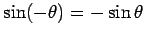. Hence, we obtain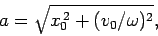(513)

and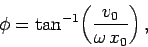(514)

since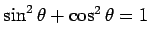and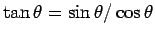.

The kinetic energy of the system is written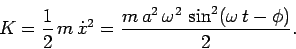(515)

Recall, from Sect. 5.6, that the potential energy takes the form(516)

Hence, the total energy can be written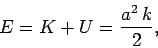(517)

sinceand. Note that the total energy is a constant of the motion, as expected for an isolated system. Moreover, the energy is proportional to the amplitude squared of the motion. It is clear, from the above expressions, that simple harmonic motion is characterized by a constant backward and forward flow of energy between kinetic and potential components. The kinetic energy attains its maximum value, and the potential energy attains it minimum value, when the displacement is zero (i.e., when). Likewise, the potential energy attains its maximum value, and the kinetic energy attains its minimum value, when the displacement is maximal (i.e., when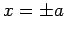). Note that the minimum value ofis zero, since the system is instantaneously at rest when the displacement is maximal.Next: The torsion pendulum Up: Oscillatory motion Previous: Introduction
Richard Fitzpatrick 2006-02-02